# Introduction to Gases n Earth is surrounded by

• Slides: 44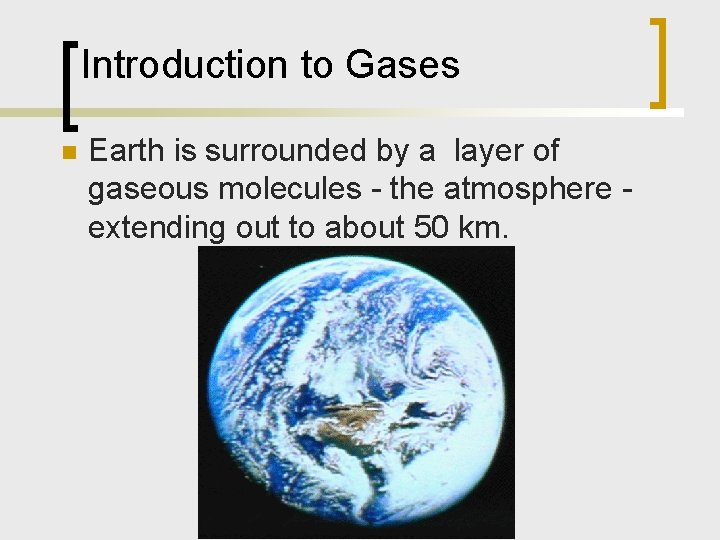Introduction to Gases n Earth is surrounded by a layer of gaseous molecules - the atmosphere extending out to about 50 km.Characteristics of Gases n Gases low density; compressible ¡ volume and shape of container ¡ expand when heated ¡ large distance between particles ¡ n Model of a gas: rapidly moving particles: vol. & shape of container 12 m 05 vd 1 ¡ no attraction between particles ¡ moving about freely ¡ large space between particles: low density & high compressibility ¡Liquids and Solids n Liquids higher density, lower compressibility ¡ characteristic volume; shape of container ¡ particles closer together; moving about; experience attractive forces ¡ n Solids high density; low compressibility ¡ particles are close together; little empty space; strong attractive forces ¡ characteristic volume and shape ¡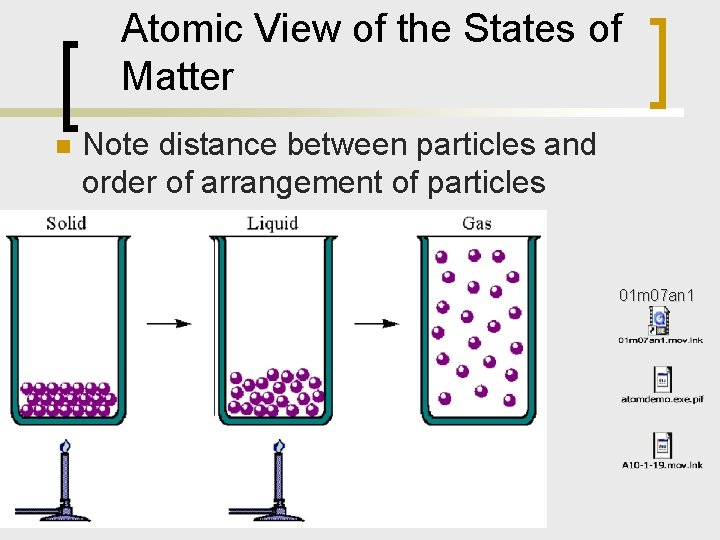Atomic View of the States of Matter n Note distance between particles and order of arrangement of particles Figure 9. 1 01 m 07 an 1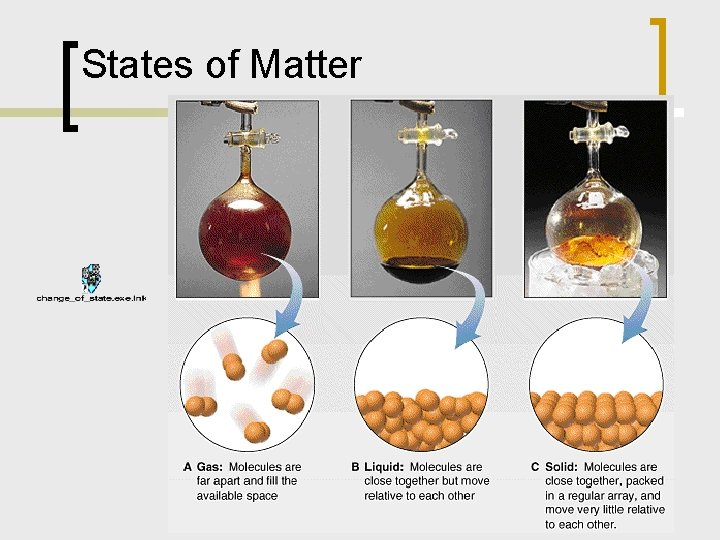States of Matter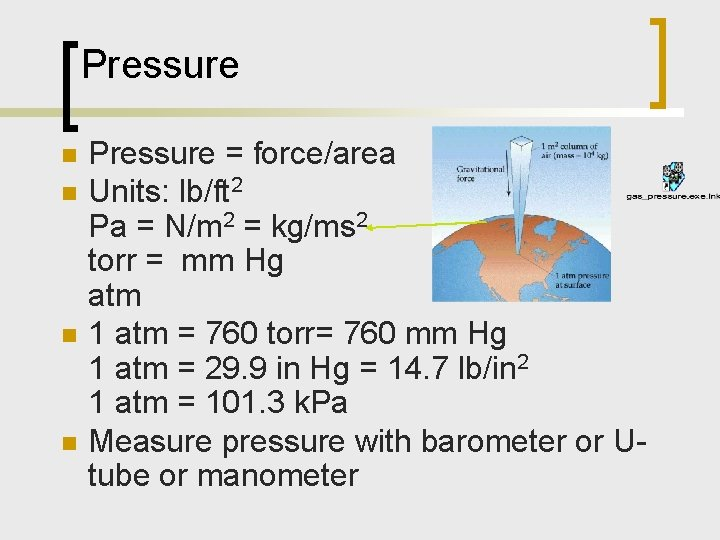Pressure n n Pressure = force/area Units: lb/ft 2 Pa = N/m 2 = kg/ms 2 torr = mm Hg atm 1 atm = 760 torr= 760 mm Hg 1 atm = 29. 9 in Hg = 14. 7 lb/in 2 1 atm = 101. 3 k. Pa Measure pressure with barometer or Utube or manometerBarometer n What is in a vacuum? What is the weight of the atmosphere? Figure 9. 3Pressure n Lab: soft drink can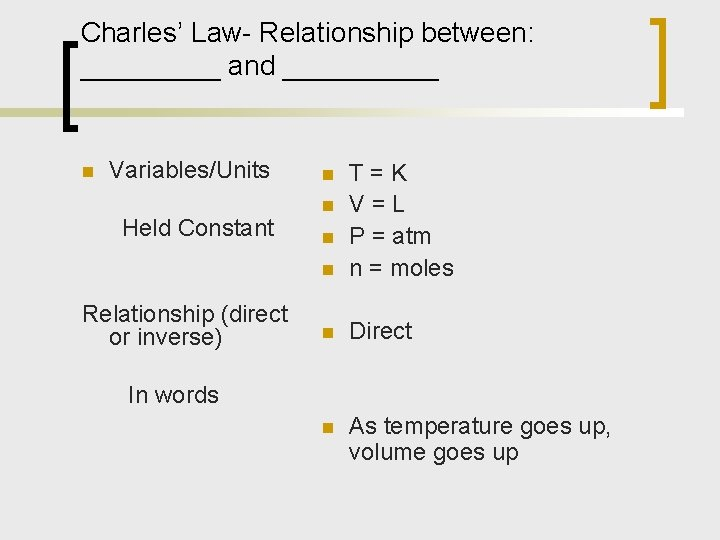Charles’ Law- Relationship between: _____ and _____ n Variables/Units Held Constant Relationship (direct or inverse) n T=K V=L P = atm n = moles n Direct n As temperature goes up, volume goes up n n n In wordsCharles’ Law n Graph of RelationshipCharles’ Law n n n As x increases, y stays the same, no relationship. y = b y is constant As x increases, y increases Y = mx + b As x increases, y decreases Y = m(1/x) + b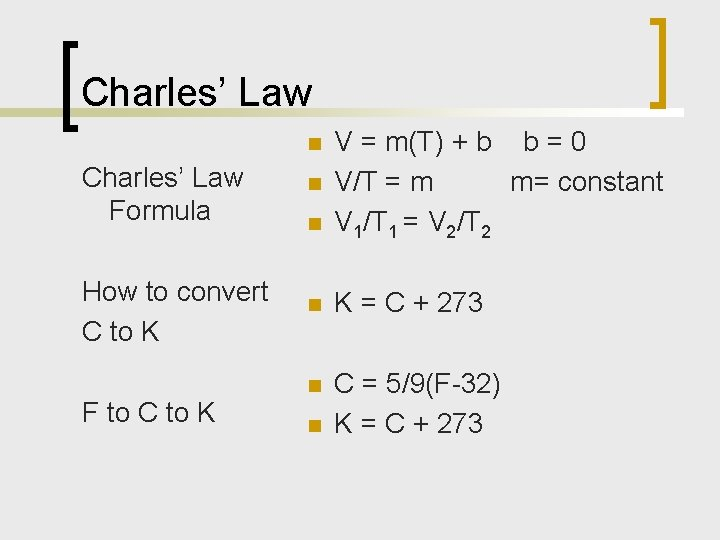Charles’ Law Formula n n V = m(T) + b b = 0 V/T = m m= constant V 1/T 1 = V 2/T 2 How to convert C to K n K = C + 273 n C = 5/9(F-32) K = C + 273 n F to C to K n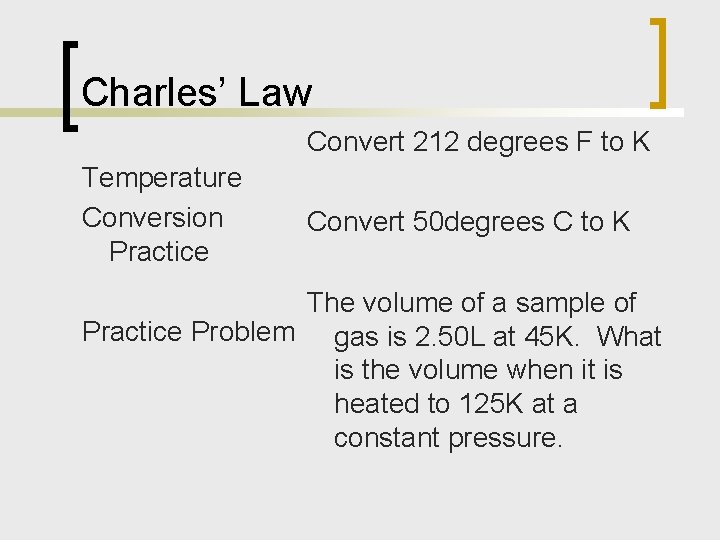Charles’ Law Convert 212 degrees F to K Temperature Conversion Practice Convert 50 degrees C to K The volume of a sample of Practice Problem gas is 2. 50 L at 45 K. What is the volume when it is heated to 125 K at a constant pressure.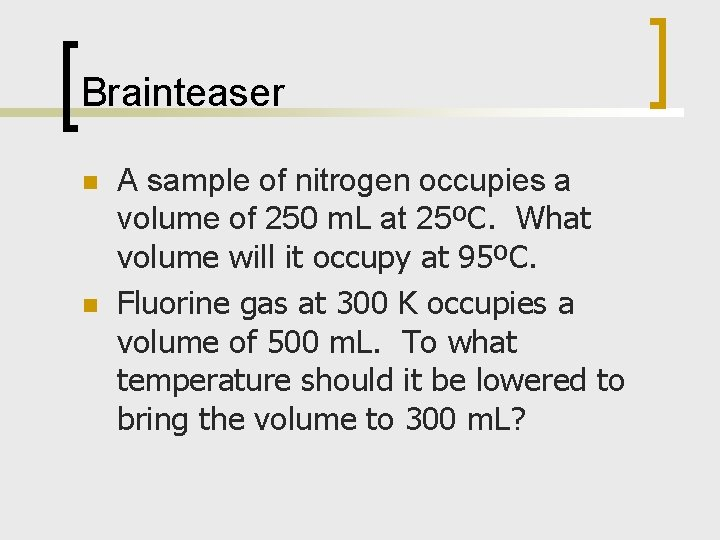Brainteaser n n A sample of nitrogen occupies a volume of 250 m. L at 25ºC. What volume will it occupy at 95ºC. Fluorine gas at 300 K occupies a volume of 500 m. L. To what temperature should it be lowered to bring the volume to 300 m. L?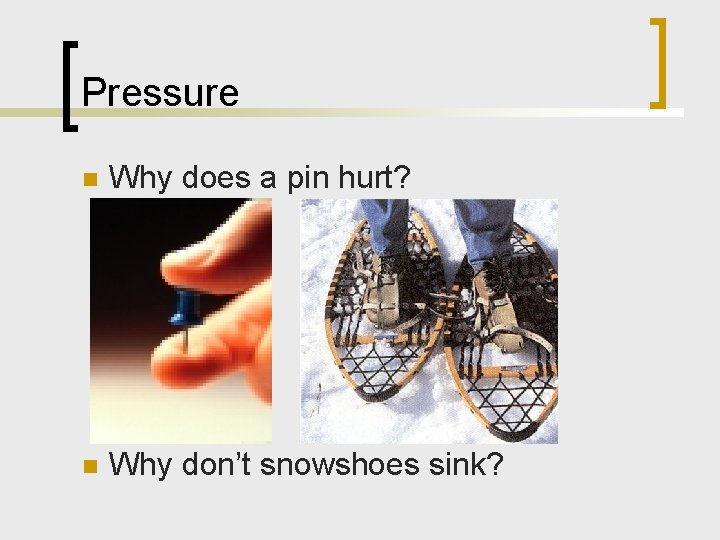Pressure n Why does a pin hurt? n Why don’t snowshoes sink?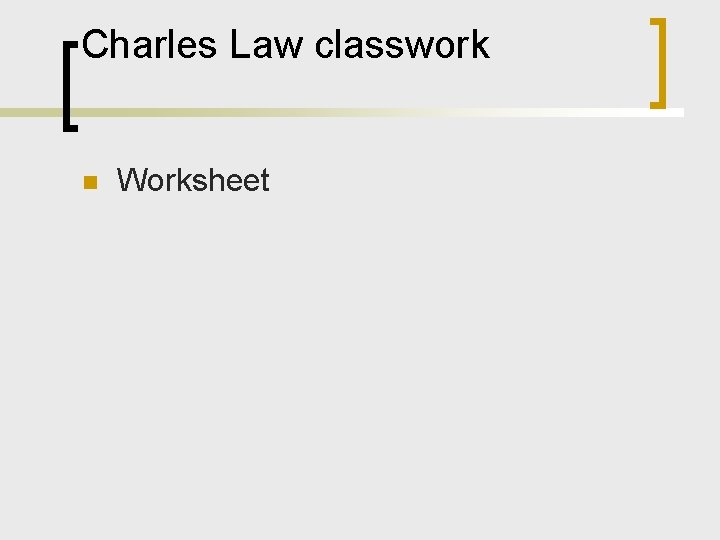Charles Law classwork n Worksheet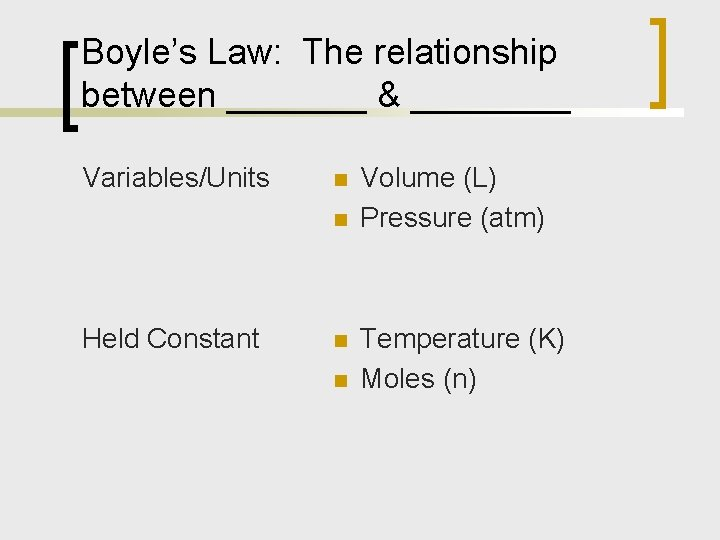Boyle’s Law: The relationship between _______ & ____ Variables/Units n n Held Constant n n Volume (L) Pressure (atm) Temperature (K) Moles (n)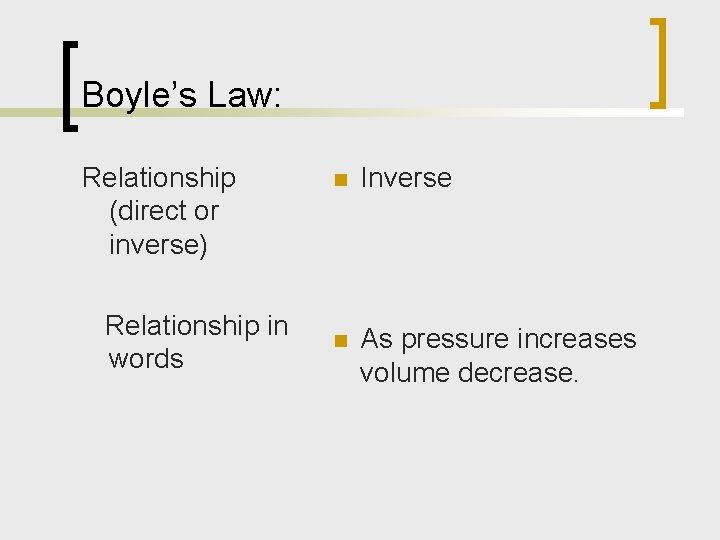Boyle’s Law: Relationship (direct or inverse) Relationship in words n Inverse n As pressure increases volume decrease.Boyle’s Law Sketch Graph Pressure vs. VolumeBoyle’s Law Sketch Graph Pressure vs. 1/ V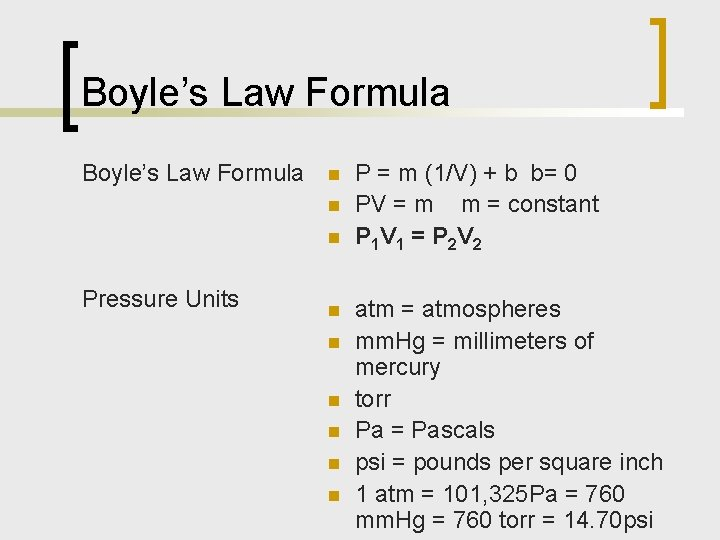Boyle’s Law Formula n n n Pressure Units n n n P = m (1/V) + b b= 0 PV = m m = constant P 1 V 1 = P 2 V 2 atm = atmospheres mm. Hg = millimeters of mercury torr Pa = Pascals psi = pounds per square inch 1 atm = 101, 325 Pa = 760 mm. Hg = 760 torr = 14. 70 psiBoyle’s Law Formula Pressure Unit Conversions n 1 atm = 101, 325 Pa = 760 mm. Hg = 760 torr = 14. 70 psiBoyle’s Law How to convert from pressure units to atm (use dimensional analysis) Convert 458 mm. Hg to atm Convert 96. 5 lb/in 2 to atm Convert 485 k. Pa to atm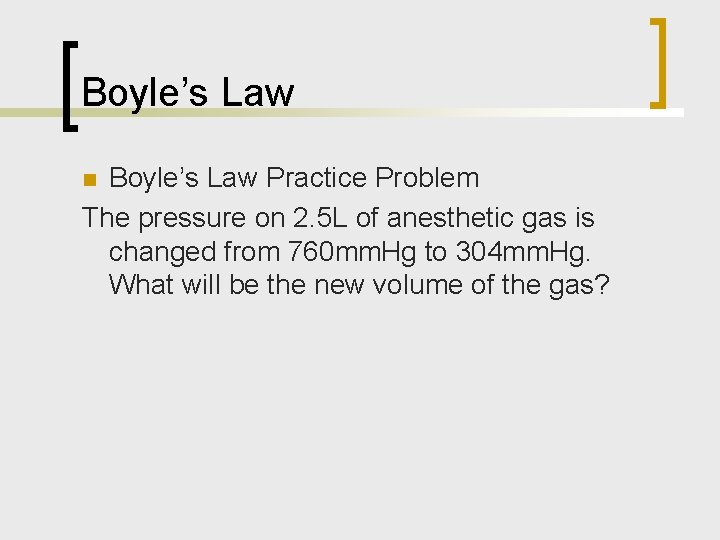Boyle’s Law Practice Problem The pressure on 2. 5 L of anesthetic gas is changed from 760 mm. Hg to 304 mm. Hg. What will be the new volume of the gas? nreview Boyle’s Law n Sulfur dioxide, a gas that plays a central role in the formation of acid rain, is found in the exhaust of automobiles and power plants. Consider a 1. 53 L sample of gaseous sulfur dioxide at a pressure of 5. 6 x 103 Pa. If the pressure changed to 0. 148 atm at a constant temperature, what will be the new volume of gas?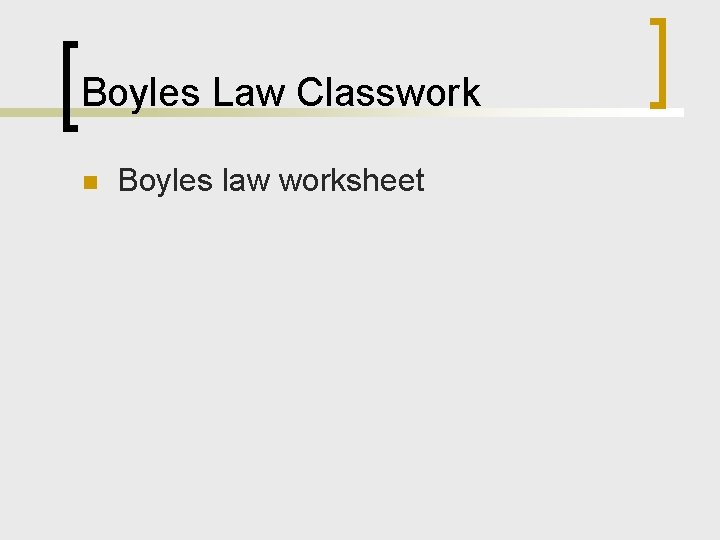Boyles Law Classwork n Boyles law worksheetUnit Conversion n Convert to the following units P = atm V = Liters T = Kelvin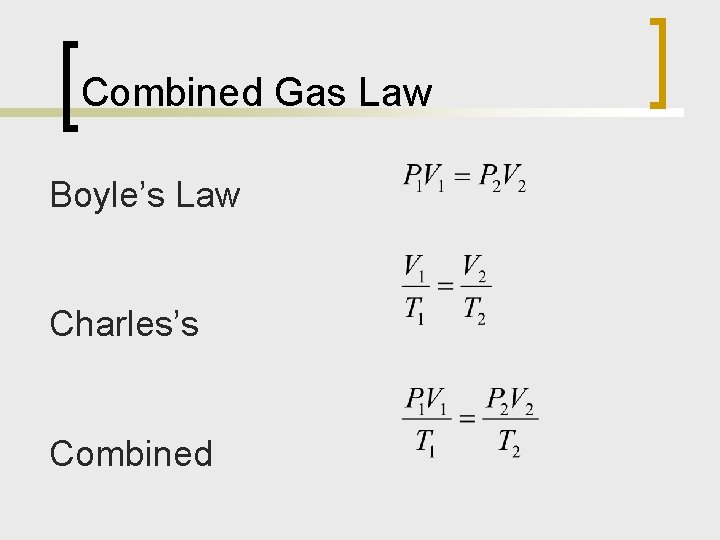Combined Gas Law Boyle’s Law Charles’s CombinedAvogadro’s Law n How can we change the volume of a gas in a balloon? Liquid N 2 balloonAvogadro’s Law n Why did the blimp deflate?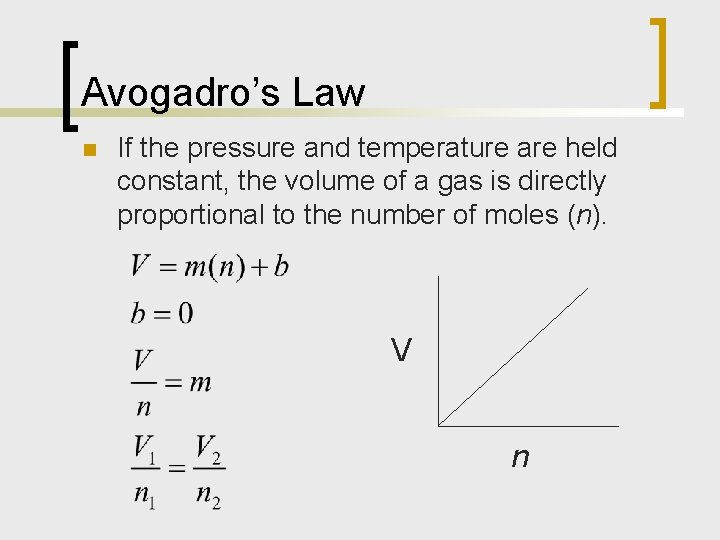Avogadro’s Law n If the pressure and temperature are held constant, the volume of a gas is directly proportional to the number of moles (n). V n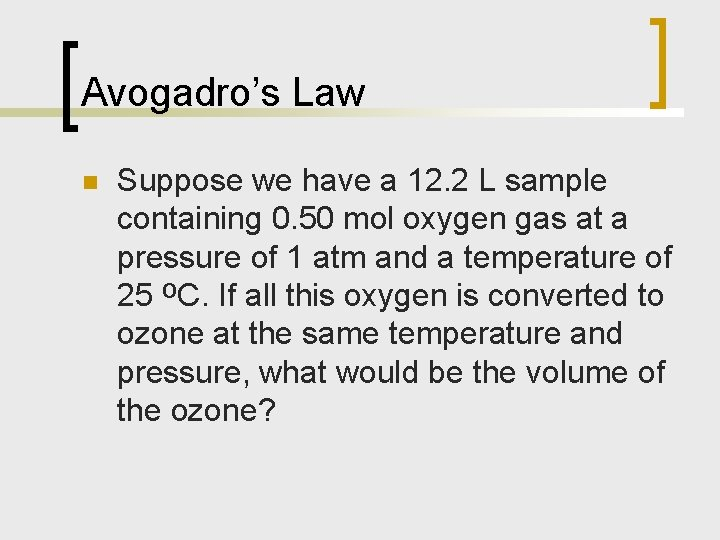Avogadro’s Law n Suppose we have a 12. 2 L sample containing 0. 50 mol oxygen gas at a pressure of 1 atm and a temperature of 25 ºC. If all this oxygen is converted to ozone at the same temperature and pressure, what would be the volume of the ozone?The Gas Laws n Variables: V, P, T, n (or m and MM or d) n Ideal Gas: properties are independent of the identity of the gas n What is the relationship between the variables for an ideal gas? Basketball Bike Tire5. 3 Ideal Gas Law n n n PV = n. RT P= pressure V = volume n = number of moles R = ideal gas constant ¡ n 0. 08206 L·atm/mol·K T = temperature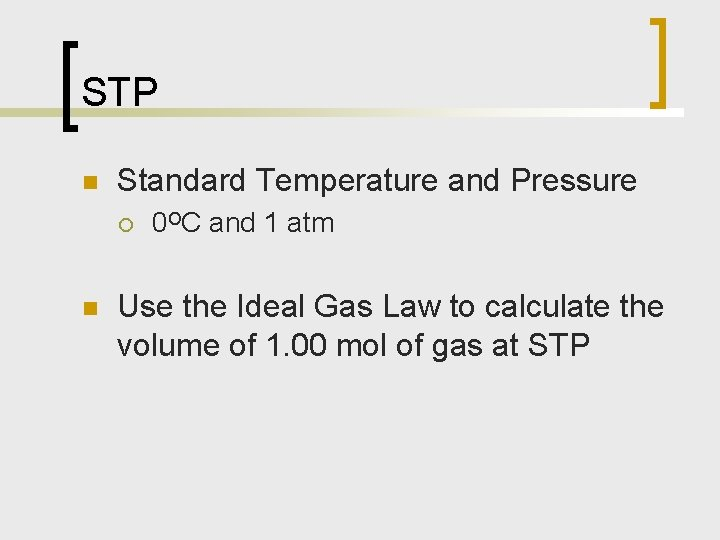STP n Standard Temperature and Pressure ¡ n 0ºC and 1 atm Use the Ideal Gas Law to calculate the volume of 1. 00 mol of gas at STPMolar Volume at STP n We can show that 22. 414 L of any gas at 0 C and 1 atm contain 6. 02 1023 gas molecules.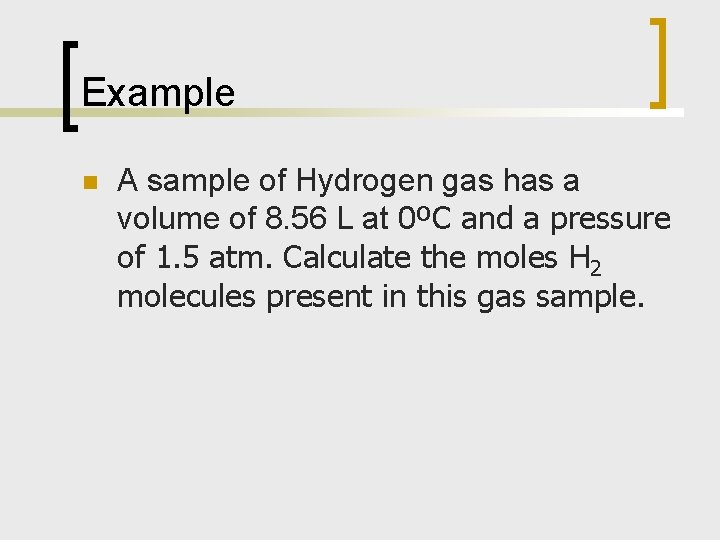Example n A sample of Hydrogen gas has a volume of 8. 56 L at 0ºC and a pressure of 1. 5 atm. Calculate the moles H 2 molecules present in this gas sample.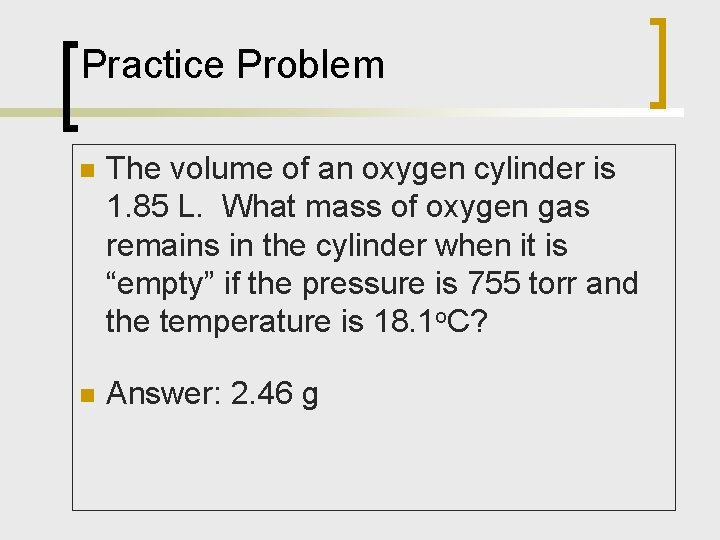Practice Problem n The volume of an oxygen cylinder is 1. 85 L. What mass of oxygen gas remains in the cylinder when it is “empty” if the pressure is 755 torr and the temperature is 18. 1 o. C? n Answer: 2. 46 gGas Law Stoichiometry n n Quicklime (Ca. O) is produced by thermal decomposition of calcium carbonate. Calculate the volume of CO 2 at STP produced from the decomposition of 152 g of Ca. CO 3 by the reaction Ca. CO 3 (s) Ca. O (s) + CO 2 (g)Molar Mass and the Ideal Gas Law n If 0. 126 g of a gas are contained in a 25 m. L tank at 25ºC and 3. 25 atm, what is the molar mass of the gas? What is the gas?Kinetic Molecular Theory n n n A model that attempts to explain the behavior of an ideal gas. The particles are so small that compared with the distances between them that the volume of the individual particles can be assumed to be negligible (zero). The particles are in constant motion. The collisions of the particles with the walls of the container are the cause of the pressure exerted by the gas.Kinetic Molecular Theory n n The particles are assumed to exert no forces on each other; they are assumed to neither attract nor repel each other. The average kinetic energy of a collection of gas particles is assumed to be directly proportional to the Kelvin temperature of the gas.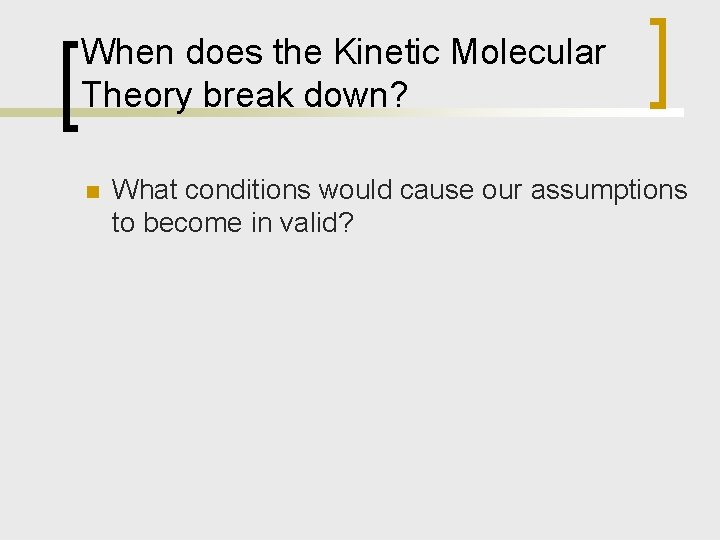When does the Kinetic Molecular Theory break down? n What conditions would cause our assumptions to become in valid?Derivations from the Ideal gas law: n n n Derivations occur in non-ideal conditions such as low temperature or high pressure: At high density the volume of the particles themselves become important. Gas particles do attract each other when they are close together.